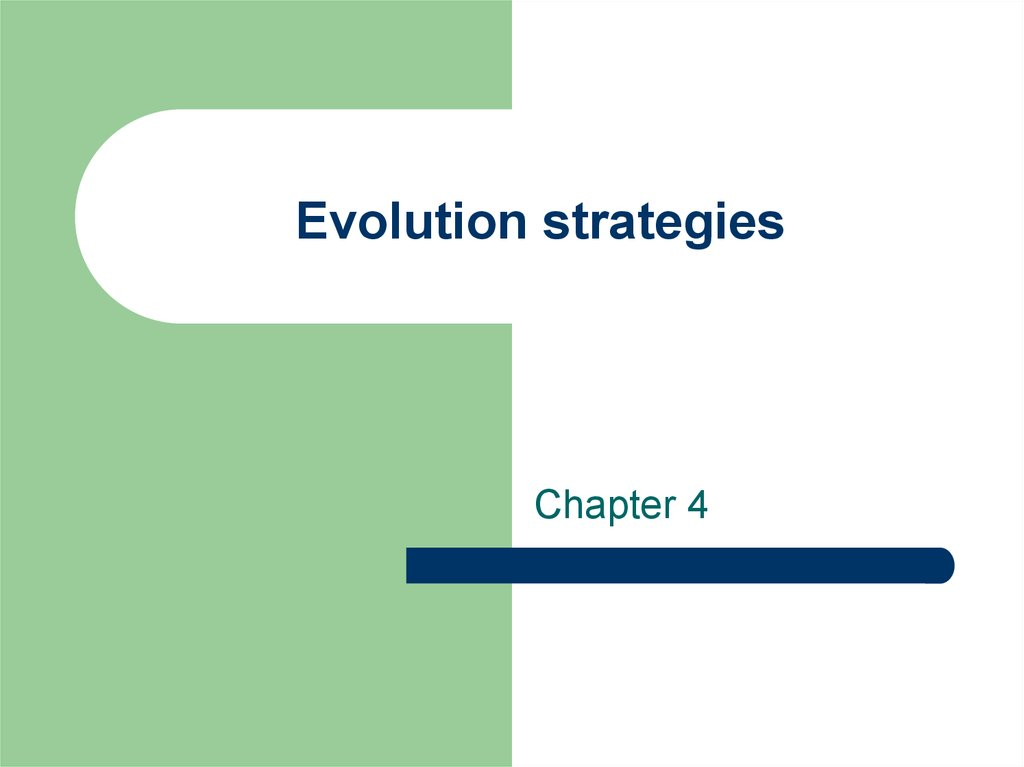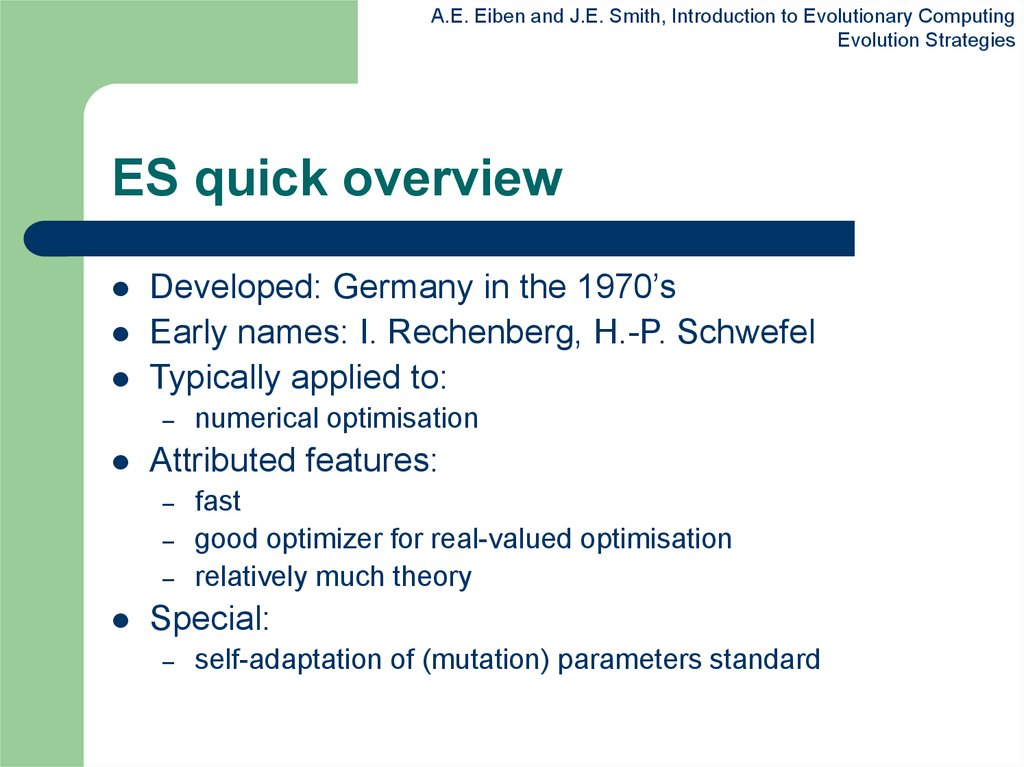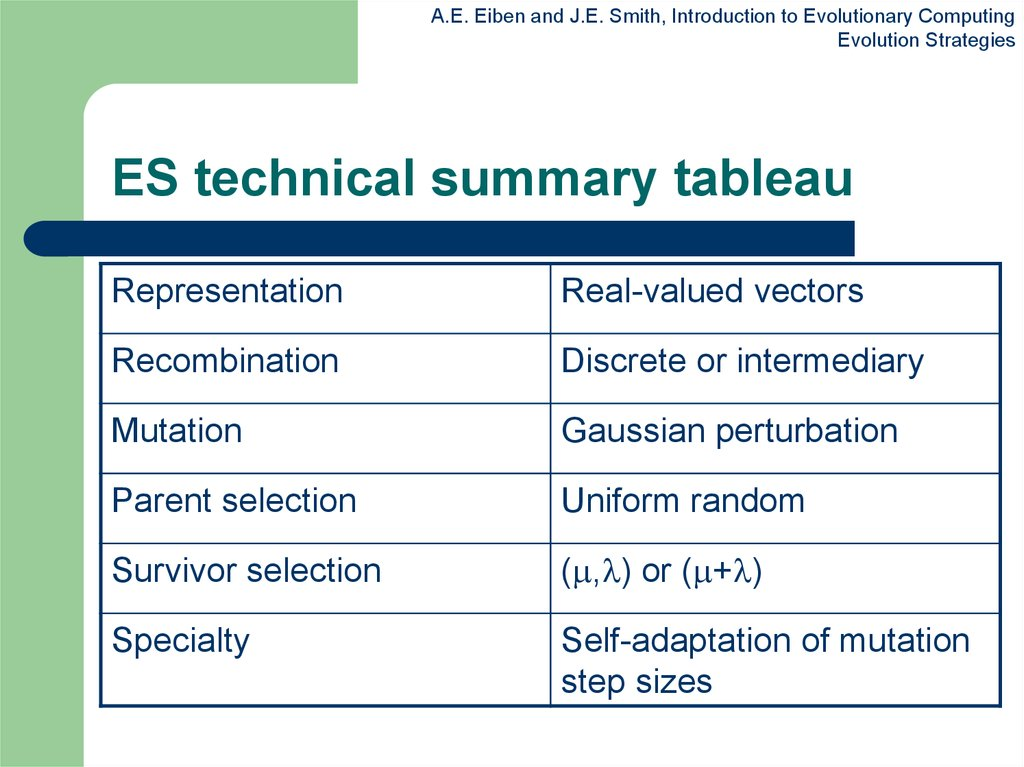# Evolution strategies. Chapter 4

Chapter 4

## 2. ES quick overview

A.E. Eiben and J.E. Smith, Introduction to Evolutionary Computing
Evolution Strategies
ES quick overview
Developed: Germany in the 1970’s
Early names: I. Rechenberg, H.-P. Schwefel
Typically applied to:

Attributed features:

numerical optimisation
fast
good optimizer for real-valued optimisation
relatively much theory
Special:

## 3. ES technical summary tableau

A.E. Eiben and J.E. Smith, Introduction to Evolutionary Computing
Evolution Strategies
ES technical summary tableau
Representation
Real-valued vectors
Recombination
Discrete or intermediary
Mutation
Gaussian perturbation
Parent selection
Uniform random
Survivor selection
( , ) or ( + )
Specialty
step sizes

## 4. Introductory example

A.E. Eiben and J.E. Smith, Introduction to Evolutionary Computing
Evolution Strategies
Introductory example
n
Task: minimimise f : R R
Algorithm: “two-membered ES” using

Vectors from Rn directly as chromosomes
Population size 1
Only mutation creating one child
Greedy selection

## 5. Introductory example: pseudocde

A.E. Eiben and J.E. Smith, Introduction to Evolutionary Computing
Evolution Strategies
Introductory example: pseudocde
Set t = 0
Create initial point xt = x1t,…,xnt
REPEAT UNTIL (TERMIN.COND satisfied) DO
Draw zi from a normal distr. for all i = 1,…,n
yit = xit + zi
IF f(xt) < f(yt) THEN xt+1 = xt

ELSE xt+1 = yt
FI
Set t = t+1
OD

## 6. Introductory example: mutation mechanism

A.E. Eiben and J.E. Smith, Introduction to Evolutionary Computing
Evolution Strategies
Introductory example: mutation
mechanism
z values drawn from normal distribution N( , )

is varied on the fly by the “1/5 success rule”:
This rule resets after every k iterations by

mean is set to 0
variation is called mutation step size
= / c if ps > 1/5
= • c if ps < 1/5
=
if ps = 1/5
where ps is the % of successful mutations, 0.8 c 1

## 7. Illustration of normal distribution

A.E. Eiben and J.E. Smith, Introduction to Evolutionary Computing
Evolution Strategies
Illustration of normal distribution

## 8. Another historical example: the jet nozzle experiment

A.E. Eiben and J.E. Smith, Introduction to Evolutionary Computing
Evolution Strategies
Another historical example:
the jet nozzle experiment
Task: to optimize the shape of a jet nozzle
Approach: random mutations to shape + selection
Initial shape
Final shape

## 9. Another historical example: the jet nozzle experiment cont’d

A.E. Eiben and J.E. Smith, Introduction to Evolutionary Computing
Evolution Strategies
Another historical example:
the jet nozzle experiment cont’d
Jet nozzle: the movie

## 10. The famous jet nozzle experiment (movie)

A.E. Eiben and J.E. Smith, Introduction to Evolutionary Computing
Evolution Strategies
The famous jet nozzle experiment (movie)

## 11. Genetic operators: mutations (2)

A.E. Eiben and J.E. Smith, Introduction to Evolutionary Computing
Evolution Strategies
Genetic operators: mutations (2)
The one
dimensional case

## 12.

A.E. Eiben and J.E. Smith, Introduction to Evolutionary Computing
Evolution Strategies
Representation
Chromosomes consist of three parts:

Object variables: x1,…,xn
Strategy parameters:
Mutation step sizes: 1,…, n
Rotation angles: 1,…, n
Not every component is always present
Full size: x1,…,xn, 1,…, n , 1,…, k
where k = n(n-1)/2 (no. of i,j pairs)

## 13. Mutation

A.E. Eiben and J.E. Smith, Introduction to Evolutionary Computing
Evolution Strategies
Mutation
Main mechanism: changing value by adding
random noise drawn from normal distribution
x’i = xi + N(0, )
Key idea:

is part of the chromosome x1,…,xn,
is also mutated into ’ (see later how)
Thus: mutation step size is coevolving with
the solution x

## 14. Mutate  first

A.E. Eiben and J.E. Smith, Introduction to Evolutionary Computing
Evolution Strategies
Mutate first
Net mutation effect: x, x’, ’
Order is important:

Rationale: new x’ , ’ is evaluated twice

first ’ (see later how)
then x x’ = x + N(0, ’)
Primary: x’ is good if f(x’) is good
Secondary: ’ is good if the x’ it created is good
Reversing mutation order this would not work

## 15. Mutation case 1: Uncorrelated mutation with one 

A.E. Eiben and J.E. Smith, Introduction to Evolutionary Computing
Evolution Strategies
Mutation case 1:
Uncorrelated mutation with one
Chromosomes: x1,…,xn,
’ = • exp( • N(0,1))
x’i = xi + ’ • N(0,1)
Typically the “learning rate” 1/ n½
And we have a boundary rule ’ < 0 ’ = 0

## 16. Mutants with equal likelihood

A.E. Eiben and J.E. Smith, Introduction to Evolutionary Computing
Evolution Strategies
Mutants with equal likelihood
Circle: mutants having the same chance to be created

## 17. Mutation case 2: Uncorrelated mutation with n ’s

A.E. Eiben and J.E. Smith, Introduction to Evolutionary Computing
Evolution Strategies
Mutation case 2:
Uncorrelated mutation with n ’s
Chromosomes: x1,…,xn, 1,…, n
’i = i • exp( ’ • N(0,1) + • Ni (0,1))
x’i = xi + ’i • Ni (0,1)
Two learning rate parmeters:

’ overall learning rate
coordinate wise learning rate
1/(2 n)½ and 1/(2 n½) ½
And i’ < 0 i’ = 0

## 18. Mutants with equal likelihood

A.E. Eiben and J.E. Smith, Introduction to Evolutionary Computing
Evolution Strategies
Mutants with equal likelihood
Ellipse: mutants having the same chance to be created

## 19. Mutation case 3: Correlated mutations

A.E. Eiben and J.E. Smith, Introduction to Evolutionary Computing
Evolution Strategies
Mutation case 3:
Correlated mutations
Chromosomes: x1,…,xn, 1,…, n , 1,…, k
where k = n • (n-1)/2
and the covariance matrix C is defined as:

cii = i2

cij = 0 if i and j are not correlated

cij = ½ • ( i2 - j2 ) • tan(2 ij) if i and j are correlated
Note the numbering / indices of the ‘s

## 20. Correlated mutations cont’d

A.E. Eiben and J.E. Smith, Introduction to Evolutionary Computing
Evolution Strategies
Correlated mutations cont’d
The mutation mechanism is then:
’i = i • exp( ’ • N(0,1) + • Ni (0,1))
’j = j + • N (0,1)
x ’ = x + N(0,C’)

x stands for the vector x1,…,xn
C’ is the covariance matrix C after mutation of the values
1/(2 n)½ and 1/(2 n½) ½ and 5°
i’ < 0 i’ = 0 and
| ’j | > ’j = ’j - 2 sign( ’j)

## 21. Mutants with equal likelihood

A.E. Eiben and J.E. Smith, Introduction to Evolutionary Computing
Evolution Strategies
Mutants with equal likelihood
Ellipse: mutants having the same chance to be created

## 22. Recombination

A.E. Eiben and J.E. Smith, Introduction to Evolutionary Computing
Evolution Strategies
Recombination
Creates one child
Acts per variable / position by either

Averaging parental values, or
Selecting one of the parental values
From two or more parents by either:

Using two selected parents to make a child
Selecting two parents for each position anew

## 23. Names of recombinations

A.E. Eiben and J.E. Smith, Introduction to Evolutionary Computing
Evolution Strategies
Names of recombinations
Two parents
Two fixed parents
selected for each i
zi = (xi + yi)/2
Local
intermediary
Global
intermediary
zi is xi or yi
chosen randomly
Local
discrete
Global
discrete

## 24. Parent selection

A.E. Eiben and J.E. Smith, Introduction to Evolutionary Computing
Evolution Strategies
Parent selection
Parents are selected by uniform random
distribution whenever an operator needs
one/some
Thus: ES parent selection is unbiased - every
individual has the same probability to be
selected
Note that in ES “parent” means a population
member (in GA’s: a population member
selected to undergo variation)

## 25. Survivor selection

A.E. Eiben and J.E. Smith, Introduction to Evolutionary Computing
Evolution Strategies
Survivor selection
Applied after creating children from the
parents by mutation and recombination
Deterministically chops off the “bad stuff”
Basis of selection is either:

The set of children only: ( , )-selection
The set of parents and children: ( + )-selection

## 26. Survivor selection cont’d

A.E. Eiben and J.E. Smith, Introduction to Evolutionary Computing
Evolution Strategies
Survivor selection cont’d
( + )-selection is an elitist strategy
( , )-selection can “forget”
Often ( , )-selection is preferred for:

Better in leaving local optima
Better in following moving optima
Using the + strategy bad values can survive in x, too long
if their host x is very fit
Selective pressure in ES is very high ( 7 • is the
common setting)

A.E. Eiben and J.E. Smith, Introduction to Evolutionary Computing
Evolution Strategies
Given a dynamically changing fitness
landscape (optimum location shifted every 200
generations)

adjust the mutation step size after every shift !

A.E. Eiben and J.E. Smith, Introduction to Evolutionary Computing
Evolution Strategies
Changes in the fitness values (left) and the mutation step sizes (right)

A.E. Eiben and J.E. Smith, Introduction to Evolutionary Computing
Evolution Strategies
> 1 to carry different strategies
> to generate offspring surplus
Not “too” strong selection, e.g., 7 •
( , )-selection to get rid of misadapted ‘s
Mixing strategy parameters by (intermediary)
recombination on them

## 30. Example application: the cherry brandy experiment

A.E. Eiben and J.E. Smith, Introduction to Evolutionary Computing
Evolution Strategies
Example application:
the cherry brandy experiment
Task to create a colour mix yielding a target colour (that
of a well known cherry brandy)
Ingredients: water + red, yellow, blue dye
Representation: w, r, y ,b no self-adaptation!
Values scaled to give a predefined total volume (30 ml)
Mutation: lo / med / hi values used with equal chance
Selection: (1,8) strategy

## 31. Example application: cherry brandy experiment cont’d

A.E. Eiben and J.E. Smith, Introduction to Evolutionary Computing
Evolution Strategies
Example application:
cherry brandy experiment cont’d
Fitness: students effectively making the mix
and comparing it with target colour
Termination criterion: student satisfied with
mixed colour
Solution is found mostly within 20 generations
Accuracy is very good

## 32. Example application: the Ackley function (Bäck et al ’93)

A.E. Eiben and J.E. Smith, Introduction to Evolutionary Computing
Evolution Strategies
Example application:
the Ackley function (Bäck et al ’93)
The Ackley function (here used with n =30):
1 n 2
f ( x) 20 exp 0.2
xi
n i 1
1 n
exp cos( 2 xi ) 20 e
n i 1
Evolution strategy:

Representation:

-30 < xi < 30 (coincidence of 30’s!)
30 step sizes
(30,200) selection
Termination : after 200000 fitness evaluations
Results: average best solution is 7.48 • 10 –8 (very good)